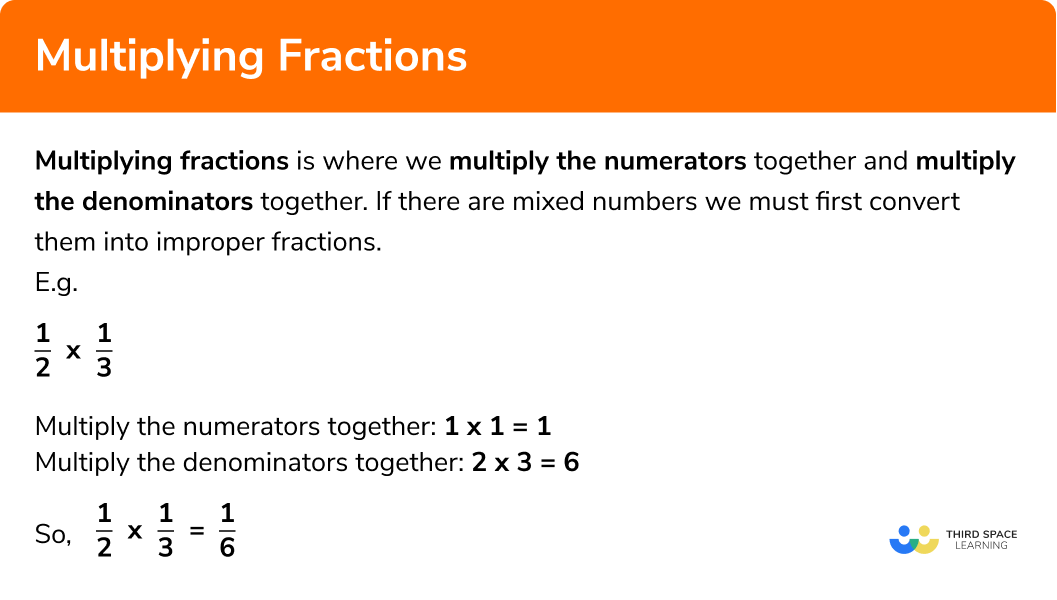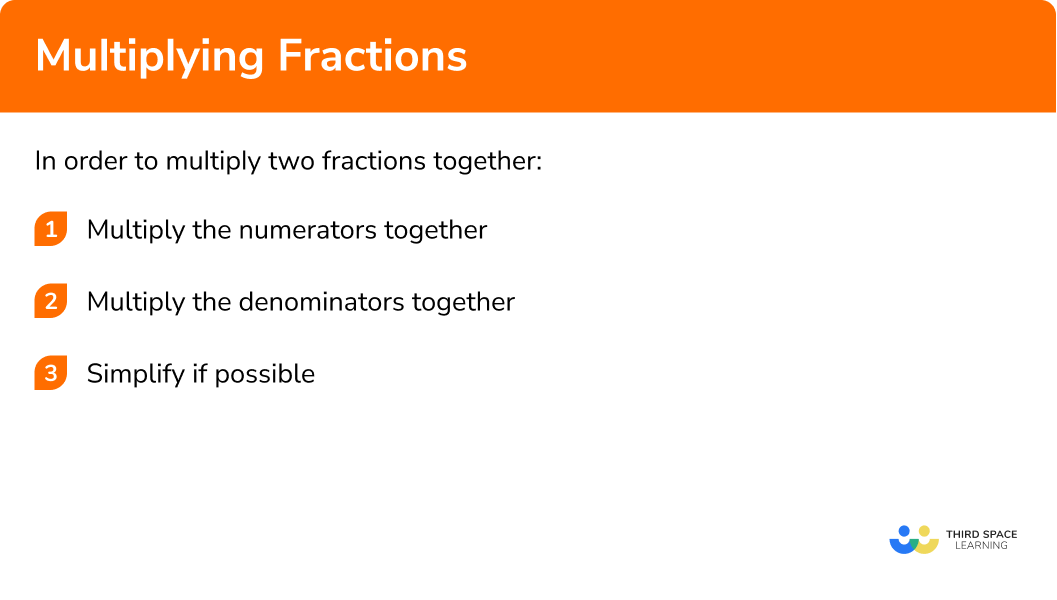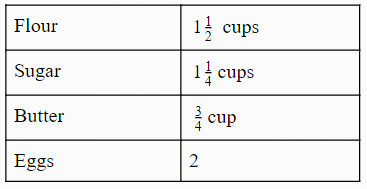# Multiplying Fractions

Here we will learn about multiplying fractions including how to multiply fractions together, multiply fractions by whole numbers, and multiply mixed fractions.

There are also multiplying fractions worksheets based on Edexcel, AQA and OCR exam questions, along with further guidance on where to go next if you’re still stuck.

## What is multiplying fractions?

Multiplying fractions is where we multiply the numerators together and multiply the denominators together. If there are mixed numbers we must first convert them into improper fractions.

Products of fractions

In order to multiply two proper fractions together:

E.g. Multiply two proper fractions

\frac{1}{2} x \frac{1}{3}

1. Multiply the numerators together: \pmb{1}x\pmb{1=1}
2. Multiply the denominators together: \pmb{2}x\pmb{3=6}
3. Simplify if possible: \pmb{\frac{1}{6}}.

In order to multiply a fraction by a whole number:

E.g. Multiply a fraction by a whole number

4 x \frac{1}{3}

1. Put the whole number over 1: \pmb{\frac{4}{1} \times \frac{1}{3}}
2. Multiply the numerators together: \pmb{4}x\pmb{1=4}
3. Multiply the denominators together: \pmb{1}x\pmb{3=3}
4. Simplify if possible: \pmb{\frac{4}{3}}

In order to multiply two mixed number fractions:

E.g. Multiply two mixed number fractions

1 \frac{1}{2} x 2 \frac{1}{4}

1. Change the mixed fractions into improper fractions: \pmb{\frac{3}{2} \times \frac{9}{4}}
2. Multiply the numerators together: \pmb{3}x\pmb{9=27}
3. Multiply the denominators together: \pmb{2}x\pmb{4=8}
4. Simplify if possible: \pmb{\frac{27}{8}}

### What is multiplying fractions?## How to multiply two fractions together

In order to multiply two fractions together:

1. Multiply the numerators together.
2. Multiply the denominators together.
3. Simplify if possible.

### Explain how to multiply fractions in 3 steps### Related lessons on fractions

Multiplying fractions is part of our series of lessons to support revision on fractions. You may find it helpful to start with the main fractions lesson for a summary of what to expect, or use the step by step guides below for further detail on individual topics. Other lessons in this series include:

## Multiplying fractions examples

### Example 1: multiplying two proper fractions together

Multiply the fractions below together:

$\frac{6}{7} \times \frac{4}{9}$

1. Multiply the numerators together.

$6×4=24$

2Multiply the denominators together.

$7×9=63$

3Simplify if possible.

When we we put the numerator and denominator together, we are left with:

$\frac{24}{63}$

If we divide both the numerator and denominator by 3 we get the simplified fraction:

$\frac{24 \div 3}{63 \div 3}=\frac{8}{21}$

### Example 2: multiplying two improper fractions together (worded question)

Find the area of the shape below:

To find the area of the rectangle we must use the formula:

\begin{aligned} A &=l \times w \\ &=\frac{15}{2} \times \frac{5}{4} \end{aligned}
$15×5=75$

$4×2=8$

When we we put the numerator and denominator together, we are left with:

$\frac{75}{8}$

We cannot simplify this fraction, so the final answer is:

$\frac{75}{8} m^{2}$

Note: always remember to square the units when calculating the area

### Example 3: multiplying three fractions together

Multiply the fractions below together:

$\frac{3}{7} \times \frac{4}{5} \times \frac{1}{2}$
$3×4×1=12$

$7×5×2=70$

When we we put the numerator and denominator together, we are left with:

$\frac{12}{70}$

If we divide both the numerator and denominator by 2, we get the simplified fraction:

$\frac{12 \div 2}{70 \div 2}=\frac{6}{35}$

## How to multiply fractions by a whole number

In order to multiply two fractions together:

1. Convert the whole number into a fraction by putting the number over a denominator of 1.
2. Multiply the numerators together.
3. Multiply the denominators together.
4. Simplify if possible.

## Multiplying fractions by a whole number examples

### Example 4: multiplying one proper fraction by a whole number

Multiply:

$\frac{2}{3} \times 4$

Rewrite the question as

$\frac{2}{3} \times \frac{4}{1}$

$2×4=8$

$3×1=3$

When we we put the numerator and denominator together, we are left with:

$\frac{8}{3}$

### Example 5: finding fractions of amounts

What is

$\frac{2}{9} \text { of } 45$

In this question the word ‘of ‘ can be changed into a multiplication sign.

Rewrite the question as

$\frac{2}{9} \times \frac{45}{1}$

$2×45=90$

$9×1=9$

When we we put the numerator and denominator together, we are left with:

$\frac{90}{9}$

This can be further simplified to 10 (because 90 ÷ 9 = 10).

Note: a shortcut to check your answer when dealing with fractions of amounts is to ‘divide by the denominator and times by the numerator.’

## How to multiply mixed fractions

In order to multiply two fractions together:

1. Convert the mixed numbers into improper fractions. Simplify if possible.
2. Multiply the numerators together
3. Multiply the denominators together
4. Simplify if possible

## Multiplying mixed fractions examples

### Example 6: multiplying two mixed numbers

Multiply the fractions below together:

$2 \frac{1}{4} \times 2 \frac{1}{2}$

To convert a mixed number into an improper fraction, multiply the denominator by the whole number and then add it to the numerator.

$2 \frac{+1}{\times4}=\frac{9}{4}$
$2 \frac{+1}{\times2}=\frac{5}{2}$

So now we are left with:

$\frac{9}{4} \times \frac{5}{2}$

$9×5=45$

$4×2=8$

This fraction cannot be simplified so we are left with our final answer as

$\frac{45}{8}$

### Common misconceptions

• Confusing multiplying and division multiplication rules

A common error is to mix up multiplication and division rules and accidentally flip the second fraction during a multiplication.

• Cross multiplying instead of multiplying numerators together and denominators together

E.g.

$\frac{2}{3} \times \frac{1}{4} \neq \frac{8}{3}$

• Finding the common denominator

A common error is to confuse the rules of adding and subtracting fractions with multiplying and dividing. It is not necessary to find a common denominator when multiplying fractions.

### Practice multiplying fractions questions

1. \frac{4}{11} \times \frac{1}{9}

\frac{4}{9}\frac{36}{11}\frac{47}{99}\frac{4}{99}Multiply the numerators: 4\times1=4

Multiply the denominators: 11\times9=99

2. Work out \frac{2}{7} \times \frac{6}{10} . Give your answer in its simplest form.

\frac{6}{35}\frac{3}{35}\frac{31}{35}\frac{10}{21}Multiply the numerators: 2\times6=12

Multiply the denominators: 7\times10=70

Simplify the fraction: \frac{12}{70}=\frac{6}{35}

3. \frac{2}{9} \times {5}

\frac{2}{55}\frac{10}{45}\frac{10}{11}5\frac{57}{9}First write 5 as \frac{5}{1} . Now we can work out \frac{2}{11} \times \frac{5}{1}

Multiply the numerators: 2\times5=10

Multiply the denominators: 11\times1=11

4. Work out \frac{4}{5} of 40

50\frac{160}{200}32\frac{204}{5}\frac{4}{5} of 40 is the same as \frac{4}{5} \times 40 . We can then rewrite this as \frac{4}{5} \times \frac{40}{1} .

Multiply the numerators: 4\times40=160

Multiply the denominators: 5\times1=5

Simplify the fraction: \frac{160}{5} = 32

5. Calculate 3 \frac{2}{3} \times 1 \frac{1}{5}

4 \frac{2}{5}3 \frac{2}{15}2 \frac{17}{18}2 \frac{13}{15}Before we multiply these numbers, we need to change them to improper fractions.
3 \frac{2}{3}= \frac{11}{3}\\ 1 \frac{1}{5} = \frac{6}{5}

We now need to calculate \frac{11}{3} \times \frac{6}{5}

Multiply the numerators: 11\times6=66

Multiply the denominators: 3\times5=15

Simplify the fraction: \frac{66}{15} = \frac{22}{5} = 4 \frac{2}{5}

6. The dimensions of a rectangular wall are 4 \frac{2}{5} by 1 \frac{1}{4} . One tin of paint can cover an area of 4 square metres. How many tins of paint is needed to paint the entire wall?

341012The area of a rectangle is base \times height therefore to work out the area of the wall we need to calculate 4 \frac{2}{5} \times 2 \frac{1}{4} .

To do this we need to convert the mixed numbers to improper fractions.
4 \frac{2}{5} = \frac{22}{5}\\ 2 \frac{1}{4} = \frac{9}{4}

Now we need to calculate \frac{22}{5} \times \frac{9}{4} .

Multiply the numerators: 22\times9=198

Multiply the denominators: 5\times4=20

Simplify the fraction: \frac{198}{20} = \frac{99}{10} = 9.9

We have 9.9 \mathrm{m}^{2} of wall to paint and each tin can cover 3\mathrm{m}^{2} .
So 9.9 \div 3 = 3.3

Therefore 4 tins of paint are needed to paint the wall.

### Multiplying fractions GCSE questions

1.

(a) Work out \frac{3}{5} \times \frac{2}{7}.

(b) Work out 1\frac{1}{4} \times 4 \frac{2}{9} . Express your answer in the simplest form.

(3 marks)

a)

\frac{6}{35}

Correctly multiplies numerators and denominators together.

(1)

b)

\frac{5}{4} or \frac{38}{9} seen  (converts to improper fraction)

(1)

\frac{95}{18}

(1)

2. Here is the recipe for 12 cupcakes:Lily wants to bake 30 cupcakes so she needs to do 2\frac{1}{2} times the recipe.

How many cups of sugar should Lily use?

(3 marks)

1\frac{1}{4} = \frac{5}{4} or 2 \frac{1}{2} = \frac{5}{2}

(1)

\frac{5}{4} \times  \frac{5}{2}

(1)

\frac{25}{8}

(1)

3.

(a) A cattle farmer owns a rectangular field with the dimensions \frac{3}{5} \mathrm{km} by \frac{1}{2} \mathrm{km}.

What is the area of the field?

b) Each cow requires an area of \frac{1}{250} \mathrm{km}^{2} to graze.

What is the maximum number of cows that can fit into the field?

(6 marks)

a)

\frac{3}{10}

Correctly multiplying the numerators or denominators together.

(1)

\frac{3}{10} km^{2}

(1)

b)

\frac{3}{10}\div \frac{1}{250}

(1)

\frac{3}{10} \times\frac{250}{1}

(1)

\frac{750}{10} seen.

(1)

75 cows

(1)

## Learning checklist

You have now learned how to:

• Use the multiplication operation, including formal written methods, applied to proper and improper fractions and mixed numbers

## Still stuck?

Prepare your KS4 students for maths GCSEs success with Third Space Learning. Weekly online one to one GCSE maths revision lessons delivered by expert maths tutors.

Find out more about our GCSE maths tuition programme.